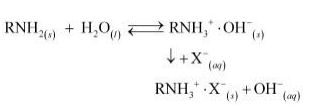9.23 Discuss the principle and method of softening of hard water by synthetic ion-exchange resins.

The process of treating permanent hardness of water using synthetic resins is based on
the exchange of cations (e.g.,  etc) and anions (e.g.,  etc)   present in water by H$*$ and OH– ions respectively.

Synthetic resins are of two types:

1) Cation exchange resins

2) Anion exchange resins

Cation exchange resins are large organic molecules that contain the $-S{O}_{3}H$ group. The resin is firstly changed to RNa (from $RS{O}_{3}H$) by treating it with NaCl. This resin then exchanges Na+ ions with $C{a}^{2+}$ and $M{g}^{2+}$ ions, thereby making the water soft.

There are cation exchange resins in H+ form. The resins exchange H+ ions for Na+, $C{a}^{2+}$, and $M{g}^{2+}$ ions.

Anion exchange resins exchange OH–ions for anions like Cl– ,$HC{O}_{3}$ , and $S{{O}_{4}}^{2-}$ present in water.During the complete process, water first passes through the cation exchange process. The water obtained after this process is free from mineral cations and is acidic in nature. This acidic water is then passed through the anion exchange process where OH–ions neutralize the H+ ions and de-ionize the water obtained.